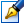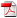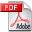##Elemente de teorie a mulţimilor în cursul preuniversitar de matematică din Germania

 Author: Robert Müller-Fonfara Degree: doctor of pedagogics Speciality: 13.00.02 - Theory and Methodology of Instruction (on disciplines) Year: 2006 Scientific adviser: Andrei Haritondoctor, professor, Tiraspol State University Institution: Scientific council:

### Status

The thesis was presented on the 7 July, 2006
Approved by NCAA on the 28 September, 2006

### Abstract0.35 Mb / in romanian

### Thesis

CZU 510.22(043.3)3.85 Mb / in romanian
141 pages

### Keywords

the modernization of mathematicians, the program, intellectual competence, experiment, curriculum, the comparative analysis, set, elements of the theory of sets, accessory, subset of the given set, association of sets, crossing of sets, difference of two sets, the binary attitude between two sets, addition between two sets, function, the law of a composition, injective function, biunique correspondence, elements of mathematical logic, logic thinking, denying of the statement, conjunction of two statements, a disjunction of two statements, implication of two statements, equivalence two statements, the single predicate, a necessary condition, mathematical operation

### Summary

The following dissertation is dedicated to the modernization of preuniversitarian mathematical education on the basis of the introduction of the elements of Theory of Sets and mathematical logic. The purpose of this study consists of the following: on the basis of analysing the process of introducing the elements of Theory of Sets and mathematical logic in the countries of Europe, with an advanced technology of instruction, such as France, England, Russia, Romania and other countries and of own work experience as the teacher of mathematics, also author of training literature on mathematics, to develop curriculum methodology of the introduction of the elements of Theory of Sets and mathematical logic in the schools of Germany. The results of the study are:

• The analysis of the introduction of the elements of Theory of sets and mathematical logic in France, England, Russia, Romania, Moldova as a result of which are obtained convincing conclusions about the effectiveness of this process, and also are noted three periods of its development:
• the active period of the introduction of the elements of Theory of sets and mathematical logic in the 1960-70's of 21st century;
• a period of moderate introduction of the elements of Theory of sets and mathematical logic (in particular, the period of stagnation in Russia) of -1980-90's of 21st century;
• the period of recovery of the values - beginning with 1990's of 21st century;
• Creation of the version of curriculum of the introduction of the elements of Theory of sets and mathematical logic for the preuniversitarian mathematical formation of Germany. Curriculum consists of five concentres and covers the entire period of preuniversitarian formation.
• a version the of the methodology of the introduction of curriculum of the introduction of the elements of Theory of sets and mathematical logic is created;
• In the dissertation study are given numerous examples (models) of the introduction of the elements of Theory of sets and mathematical logic in the preuniversitarian mathematical formation in Germany. Recommendations to which I came and suggest and realized are presented, which also will assist in the successful introduction of the elements of Theory of sets and mathematical logic in preuniversitarian and universitarian education in Germany.